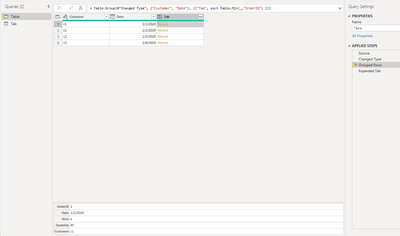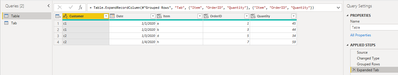cancel
Showing results for
Did you mean:## Group by and find the specific information for each group

Scenario:

Suppose I want to get the first order information for each customer in each day. How can we achieve this? Here is an example with DAX and Power Query solution.

Table Used:
Tab:Requirement:

We need to group by 'Customer' and 'Date'. And we may find the 'Item' and 'Quantity' corresponding to the first 'OrderID' in each group.

Method in DAX:

In DAX, we may create a calculated table as below.

``````Table 2 =
SUMMARIZE (
Tab,
Tab[Customer],
Tab[Date],
"Item",
VAR firstorderid =
MIN ( Tab[OrderID] )
RETURN
MAXX (
FILTER ( ALLEXCEPT ( Tab, Tab[Customer], Tab[Date] ), [OrderID] = firstorderid ),
[Item]
),
"OrderID",
VAR firstorderid =
MIN ( Tab[OrderID] )
RETURN
MAXX (
FILTER ( ALLEXCEPT ( Tab, Tab[Customer], Tab[Date] ), [OrderID] = firstorderid ),
[OrderID]
),
"Quantity",
VAR firstorderid =
MIN ( Tab[OrderID] )
RETURN
MAXX (
FILTER ( ALLEXCEPT ( Tab, Tab[Customer], Tab[Date] ), [OrderID] = firstorderid ),
[Quantity]
)
) ``````

We need to follow below three steps to get the result.

1. Group by 'Customer' and 'Date'.
``````SUMMARIZE (
Tab,
Tab[Customer],
Tab[Date],
...
...
)``````

1. Get the first 'OrderID' in each group.
``VAR firstorderid = MIN ( Tab[OrderID] ) ``

1. Find the 'Item' and 'Quantity' corresponding to the first 'OrderID' in each group.
``````MAXX (
FILTER ( ALLEXCEPT ( Tab, Tab[Customer], Tab[Date] ), [OrderID] = firstorderid ),
[Item]
) ``````

``````MAXX (
FILTER ( ALLEXCEPT ( Tab, Tab[Customer], Tab[Date] ), [OrderID] = firstorderid ),
[OrderID]
) ``````

``````MAXX (
FILTER ( ALLEXCEPT ( Tab, Tab[Customer], Tab[Date] ), [OrderID] = firstorderid ),
[Quantity]
) ``````

Result:Method in Power Query:

You can add two new step with below m codes.

``````#‘Grouped Rows’
=Table.Group(#"Changed Type", {"Customer", "Date"}, {{"Tab", each Table.Min(_,"OrderID") }}) ``````

``````#'Expanded Tab'=
Table.ExpandRecordColumn(#"Grouped Rows", "Tab", {"Item", "OrderID", "Quantity"}, {"Item", "OrderID", "Quantity"}) ``````

The steps are the same with DAX method but in different language.

1. Group by 'Customer' and 'Date', and then get the record where the 'OrderID' is minimum in the corresponding group.1. Expand the record to show the corresponding 'Item' and 'Quantity'.Conclusion:

The above scenario is often used in finding the corresponding information of the corresponding value in each group. For example, we want to get the information of the first check-in person in each project group every day. Or we'd like to know the person who does best in specific KPI in each month.